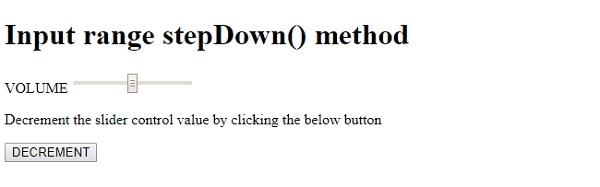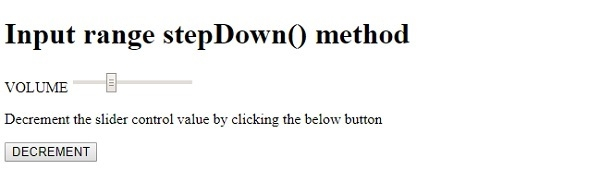HTML DOM Input Range stepDown() method

HTMLWeb DevelopmentFront End Technology

The HTML DOM Input Range stepDown() method is used for decrementing the slider control value by a specified number. If left blank then it will decrement by 1. If the step attribute is specified then the stepdown() method will decrement in multiple of that. Eg: If step=”20” then stepDown(2) will decrement by 20*2=40

Syntax

Following is the syntax for Range stepDown() method −

rangeObject.stepDown(number)

Here, number is a mandatory parameter for specifying the slider control decrement value. If left blank it will decrement by 1.

Example

Let us look at an example for the range stepDown() method −

Live Demo

<!DOCTYPE html>
<html>
<body>
<h1>Input range stepDown() method</h1>
<form>
VOLUME <input type="range" id="RANGE1" name="VOL">
</form>
<p>Decrement the slider control value by clicking the below button</p>
<button type="button" onclick="stepDecrement()">DECREMENT</button>
<p id="Sample"></p>
<script>
function stepDecrement() {
document.getElementById("RANGE1").stepDown(10);
}
</script>
</body>
</html>

Output

This will produce the following output −On clicking the DECREMENT button −In the above example −

We have created an input field contained inside a form having type=“range, id=“RANGE1” ,name=“VOL” −

<form>
VOLUME <input type="range" id="RANGE1" name="VOL">
<form>

We have then created a button DECREMENT that will execute the stepDecrement() method when clicked by the user −

<button type="button" onclick="stepDecrement()">DECREMENT</button>

The stepDecrement() method uses the getElementById() method to get the input field with type range by passing the id “RANGE1” to it and calls the stepDown() method on it with 10 supplied as decrement value. This will decrement the slider by 10 −

function stepDecrement() {
document.getElementById("RANGE1").stepDown(10);
}